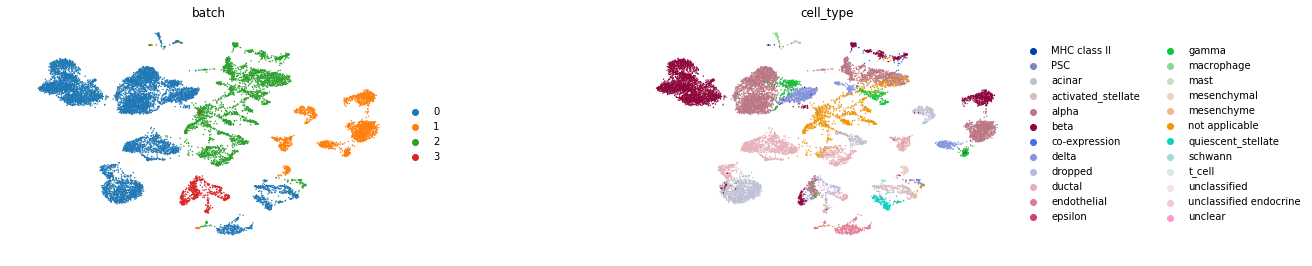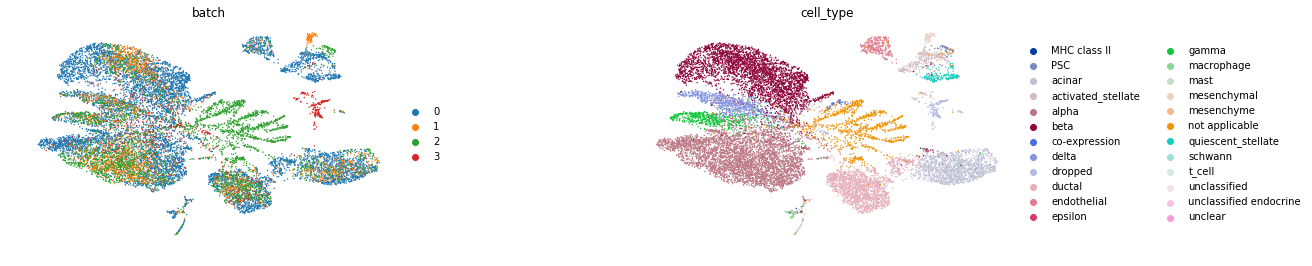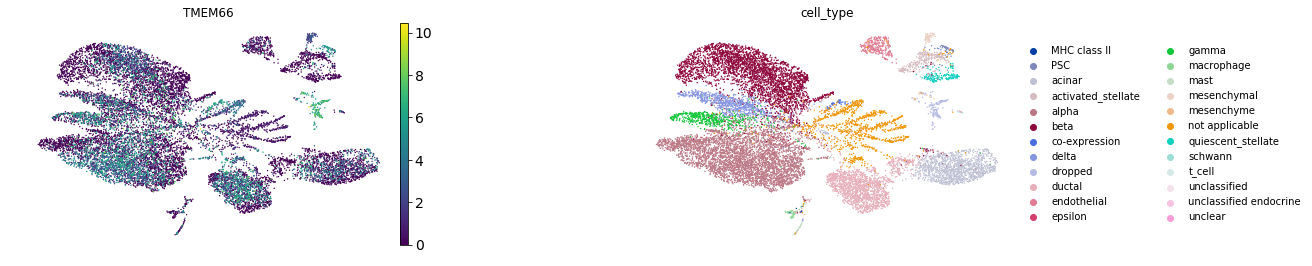In [ ]:
import scgen
import scanpy as sc


In [ ]:
train = sc.read("./tests/data/pancreas.h5ad",


We need two observation labels "batch" and "cell_type" for our batch_removal procedure. There exist a "batch" obs but no "cell_type", so we add it as a .obs of adata

In [ ]:
train.obs["cell_type"] = train.obs["celltype"].tolist()


### UMAP Visualization of the original uncorrected data¶

In [ ]:
sc.pp.neighbors(train)
sc.tl.umap(train)

In :
sc.pl.umap(train, color=["batch", "cell_type"], wspace=.5, frameon=False)### Creating and training the model¶

In :
network = scgen.VAEArith(x_dimension= train.shape, model_path="./models/batch" )


We train the model for 100 epochs

In :
network.train(train_data=train, n_epochs=100)


### Batch Removal¶

Now, we pass our train (uncorected) adata and network object to batch_removal fucntion which returns adata of batch corrected data

In [ ]:
corrected_adata =  scgen.batch_removal(network, train, batch_key="batch", cell_label_key="cell_type")


### Visualization of the corrected data¶

In :
sc.pp.neighbors(corrected_adata)

WARNING: You’re trying to run this on 2448 dimensions of .X, if you really want this, set use_rep='X'.
Falling back to preprocessing with sc.pp.pca and default params.

In :
sc.pl.umap(corrected_adata, color=["batch", "cell_type"], wspace=.5, frameon=False)#### Using uncorrected data¶

Note that original adata.raw for the adata.raw is saved to corrected_adata.raw and you can use that for fruther analaysis

In :
corrected_adata.raw

Out:
<anndata.core.anndata.Raw at 0x7f4da4313390>
In :
sc.pl.umap(corrected_adata, color=["TMEM66", "cell_type"], wspace=.5, frameon=False, use_raw=True)